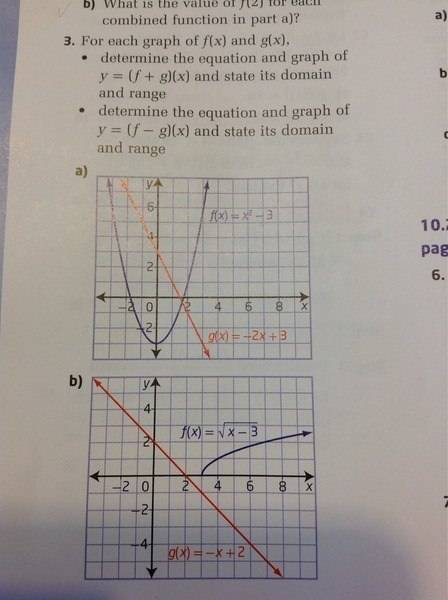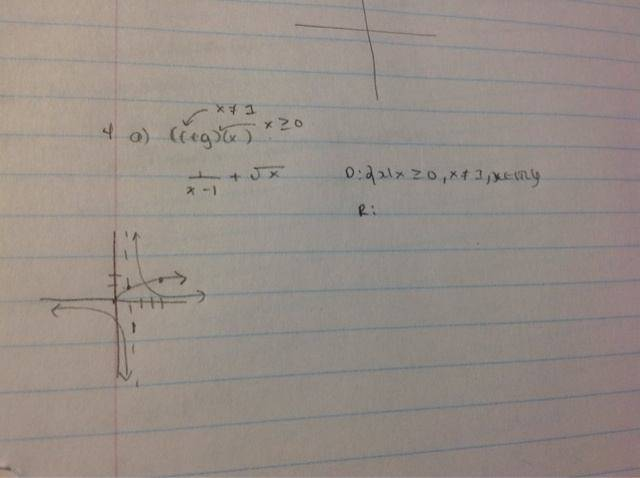# Finding domain and ranges for composition function

Coco12

## Homework Statement

Find the domain and range of (f+g)(x)
Given that
F(x)= 1/(x-1) and g(x) = sqrt(x)

## The Attempt at a Solution

I added the two functions to get 1/(x-1) + sqrt(x)

To get domain, i look at the restrictive x values for both to get x greater than 0 and not equal to 1.
However how do i find the range?

Last edited:

Mentor

## Homework Statement

Find the domain and range of (f+g)(x)
Given that
F(x)= 1/(x-1) and g(x) = sqrt(x)

Another question lets say:
f(x) = x+3
G(x)= -x-4
Find the domain and range of (f*g)(x)

## The Attempt at a Solution

I added the two functions to get 1/(x-1) + sqrt(x)

To get domain, i look at the restrictive x values for both to get x greater than 0 and not equal to 1.
However how do i find the range?
Graph both functions separately, preferably using different colors for each graph. Then, using a third color, or a marker pen, go over the parts of the two graphs for which x ≥ 0 and x ≠ 1. From that graph it should be evident what the range of the sum function is.
2) I multiplied f(x) and g(x) together to get -x^2-7x-12
My domain I got all real numbers, again how do i get the range?
The graph of the product function is a parabola that opens downward. You should already have a technique for finding the vertex of a parabola. Let's say that the vertex is at (a, b). Then the range will be {y| -∞ < y ≤ b}.

BTW, you misposted this problem in one of the physics sections. This is a precalc type problem, so I moved it to this section.

Coco12
is there another way to find the range without graphing it?
the answer is y less than -0.7886 or y greater than 2.2287.
How can you get to that precise of an answer when graphing it by hand??

Mentor
They're probably using a graphing calculator or some graphing utility.

Coco12For the second graph (f+g)(x)
The range in the back of the book says y less than .75 but u can see clearly in the graph that it is less than 1 ... What is going on?

Coco12
They're probably using a graphing calculator or some graphing utility.I drew the graph out it is just a sketch and would not be accurate on the range.. Are you expected to know precisely where it is?

Mentor
View attachment 64817
For the second graph (f+g)(x)
The range in the back of the book says y less than .75 but u can see clearly in the graph that it is less than 1 ... What is going on?
The graphs don't show the sum or difference of the two functions. Those (sum or difference) are the graphs of interest here.

Coco12
The graphs don't show the sum or difference of the two functions. Those (sum or difference) are the graphs of interest here.

The thing is how do you graph 1/(x-1) + sqrt (x)?

Mentor
You can get a rough graph by doing it by hand. Pick values of x there are in the common domain for both functions (x ≥ 0, and x ≠ 1), the calculate the y-values.

As I said before, they are probably using a graphing calculator or computer graphics package.

Coco12
So for example I would take 0.5 and plug it into the composite formula?

Coco12
But the range won't be accurate right?